## Week 6: Sum of 3 Cubes

Week 6: Sum of 3 Cubes

(a) How many of the following numbers in the table can you form as the sum of 3 cubes of integers?  (You can use negative integers if you wish).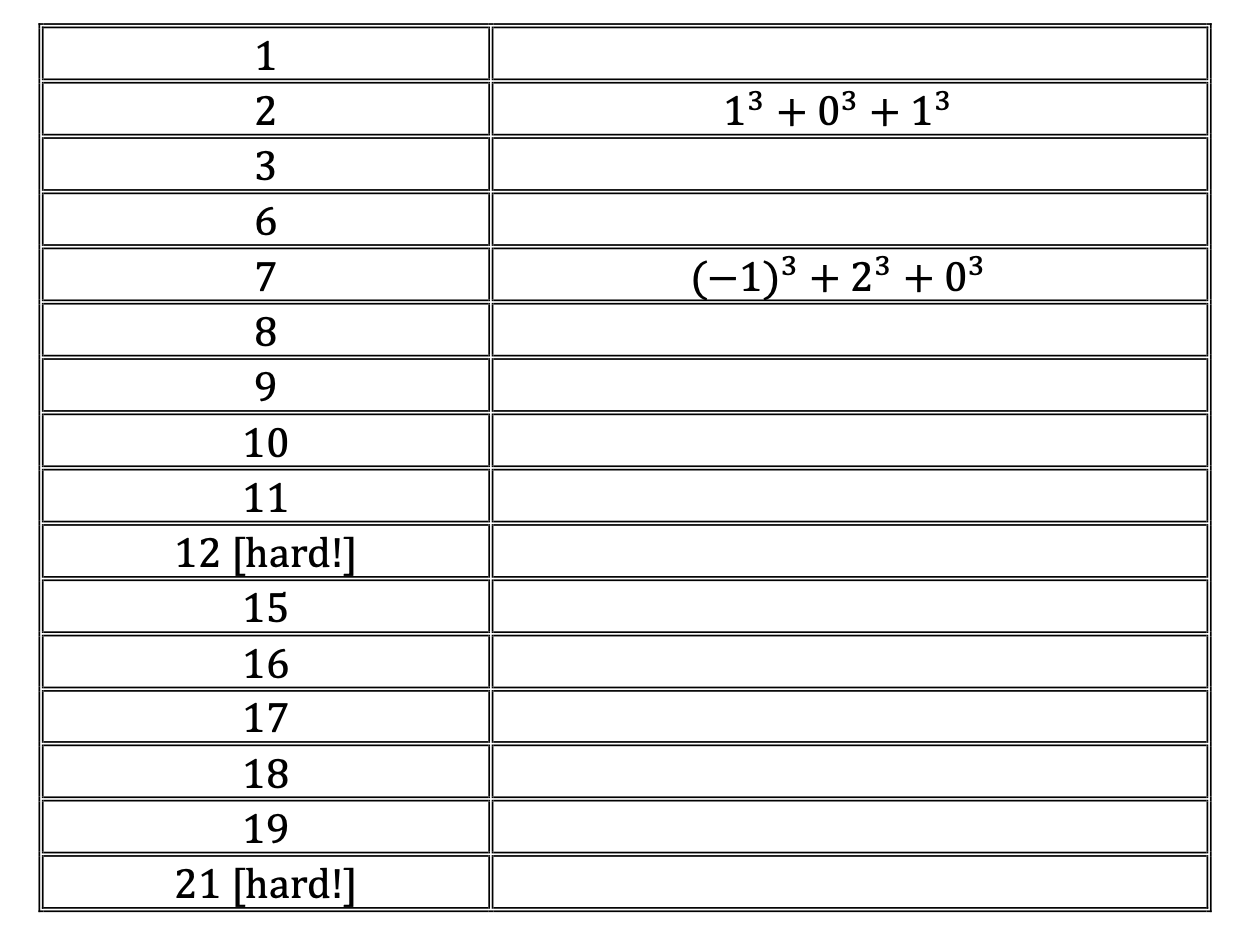(b)  Research what the solution is for 30 and 33!

(c)  Can you find a pattern that describes the numbers that are missing from this list?

Teacher notes:

Level of difficulty: Accessible for all

Syllabus knowledge required: None

This would be a good starter which could either be done when studying proof in Analysis.

## Week 5: Circular Logic

A shape is formed as follows:

A circle is drawn such that the points A, B, C, D are equally spaced around the circumference.  The arcs AB and CD are reflected in the lines AB and CD respectively.

This makes the following shape:(a)       Find the area of the shaded shape when the radius, r, of the original circle is 1.

(b)       What is the area of the shaded shape when r = a?

Teacher notes:

Level of difficulty:  Accessible for SL and HL students

Syllabus knowledge required:  Sectors and segments

This would be a good starter which can be done as a non-calculator exercise.

Solutions:

## Week 4: Decidedly Average

In the following grids, each blank square should be filled with the mean of the two numbers in adjacent squares.

(a)        Fill in the grid below.(b)       Find x in the following grid, leaving your answer in terms of a,c: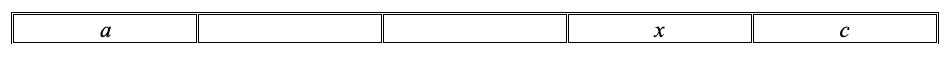(c)        Find x in the following grid, leaving your answer in terms of a,c: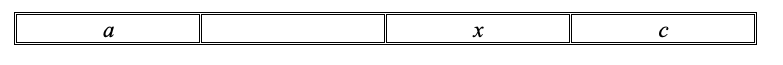(d)       For a grid of length n with first box a and last box c, make a hypothesis for the value of x, the box adjacent to c in terms of a,c,n.(e)        Check your hypothesis works when a = 10, b = 15, n = 6.

Teacher notes:

Level of difficulty:  Accessible for good SL and HL students

Syllabus knowledge required:  Basic averages and algebraic manipulation

This could be done when doing mean, median mode.

Solutions:

## Week 3: Make 2023

Using the numbers 10,9,8,7,6,5,4,3,2,1 in order, the standard arithmetic operations and brackets, can you make the number 2023?

For example of the idea: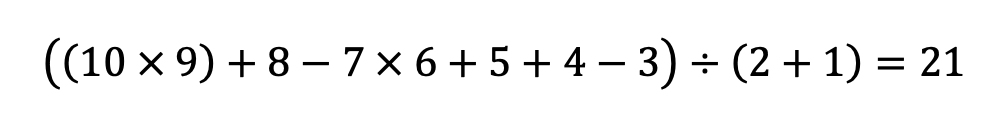This clearly doesn’t make the correct answer – but can you find a combination that does?

Teacher notes:

Level of difficulty:  Accessible for all students

Syllabus knowledge required:  none.

This would be a good starter for the start of 2023!

Solutions:

One possible solution is:Can you find any more?

## Week 2: Message in the logs

Can you find the hidden message?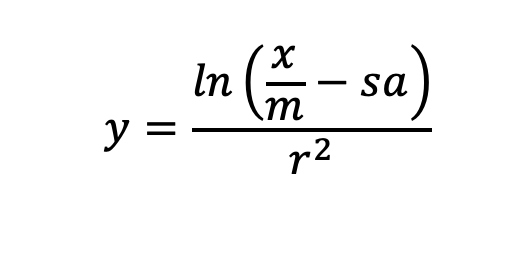Teacher notes:

Level of difficulty:  Accessible for SL Analysis and all HL students

Syllabus knowledge required:  Laws of logs

This would be a good starter in December!

Solutions:

Using the laws of logs we get:Happy Christmas!

## Week 1: Square Dance

Week 1: Square Dance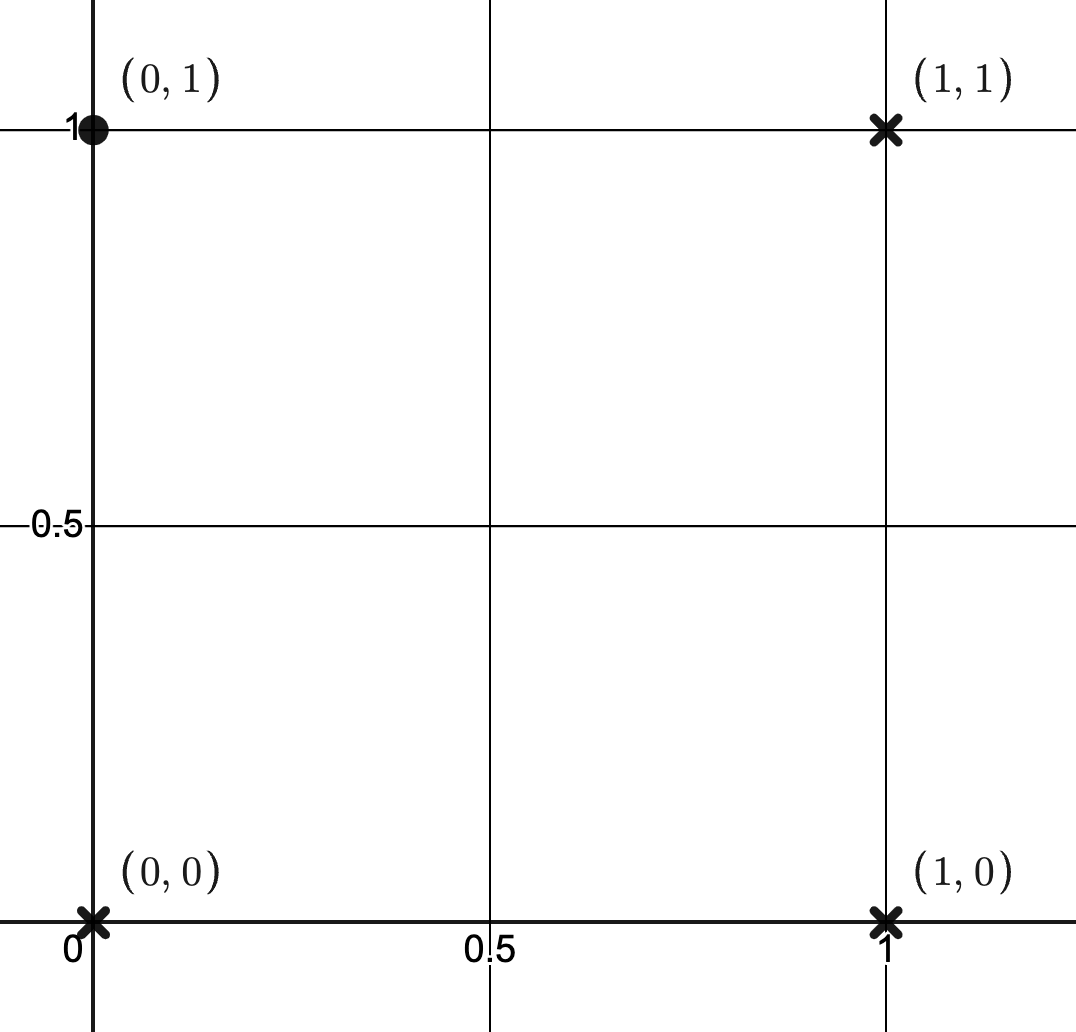This puzzle is as follows:

For the unit square shown above with vertices at (0,0), (1,0), (0,1) and (1,1), how many different types of functions can you find that go through at least 2 points?

Teacher notes:

Level of difficulty:  Accessible for strong SL and all HL students.

Syllabus knowledge required:  Functions and transformations and/or regression techniques.

This would be a good starter which could either be done as a non GDC exercise, or using Desmos regression depending on what syllabus point you intend to support.  It would be a useful exercise to support the coursework.

Solutions:

There are clearly an unlimited number of solutions.  Here are a few: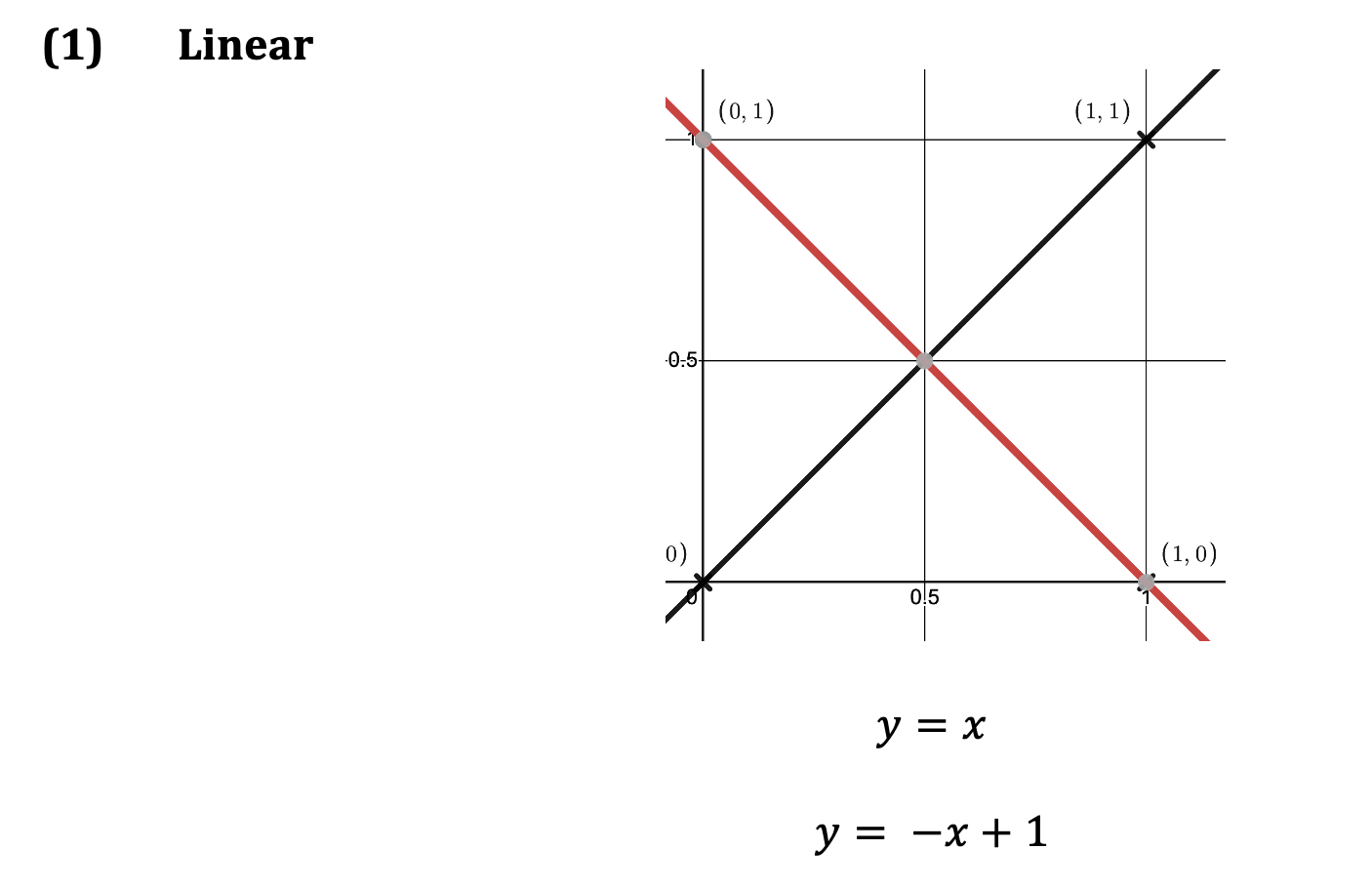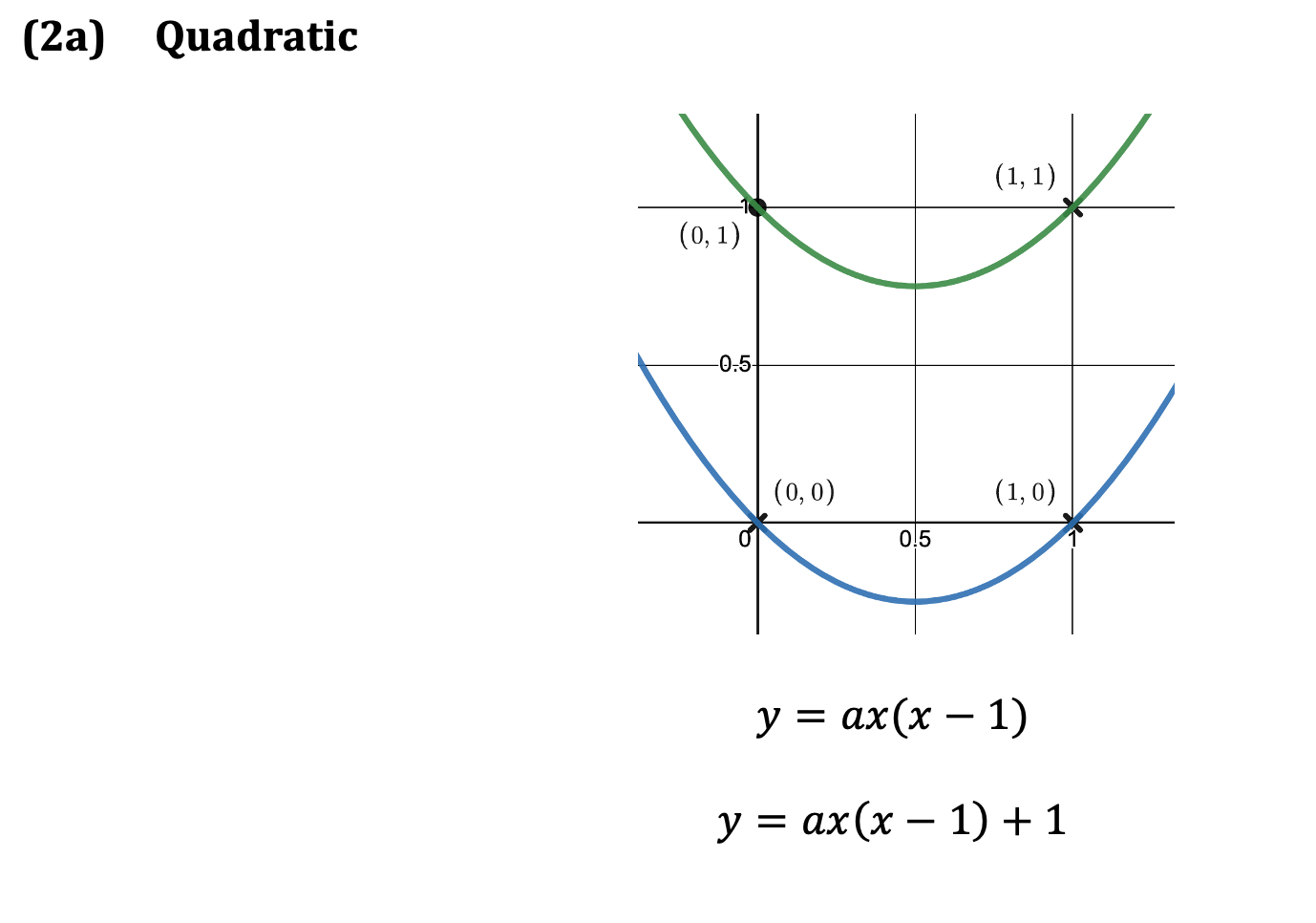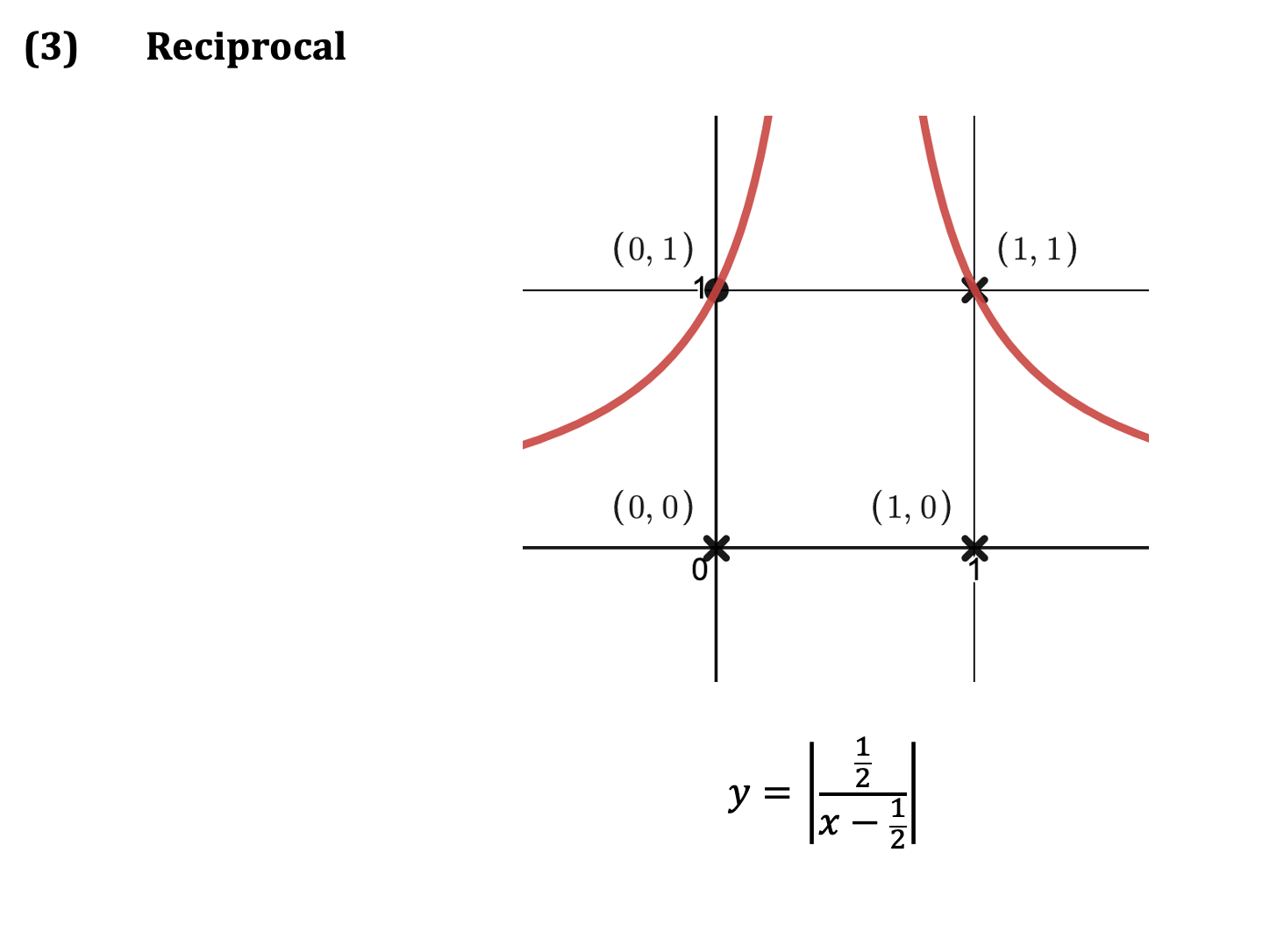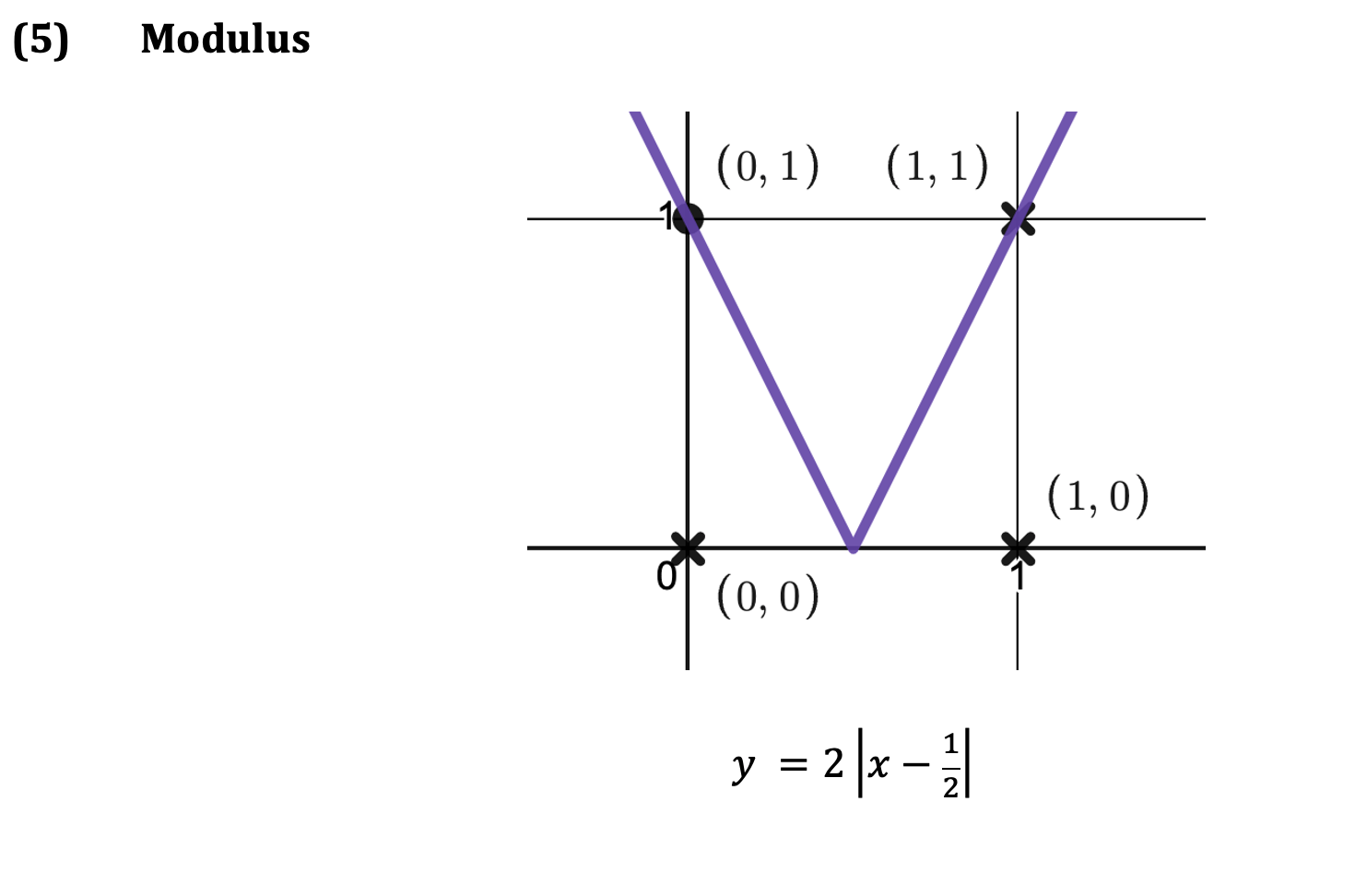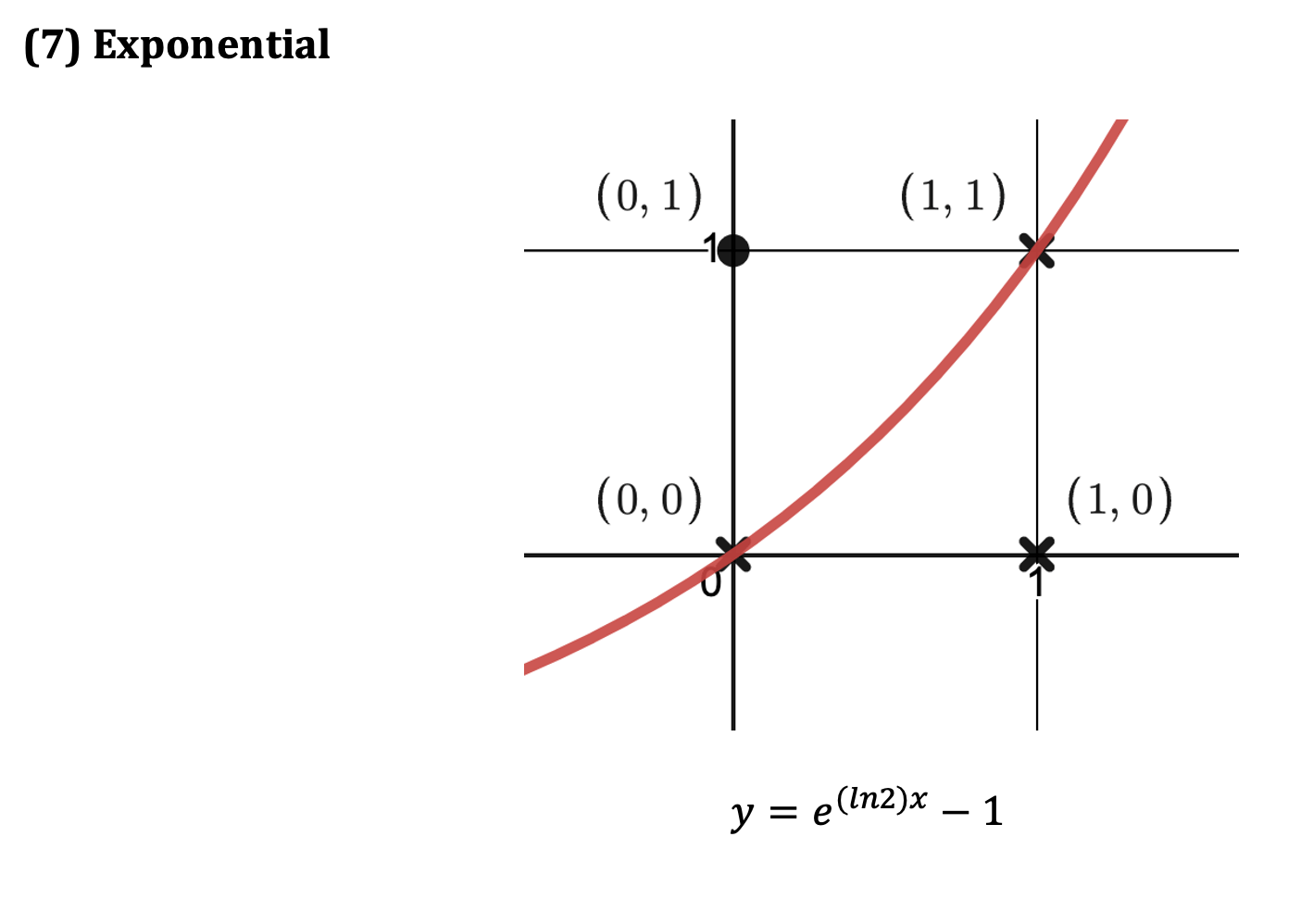You can download a pdf of the problem and the solution here.